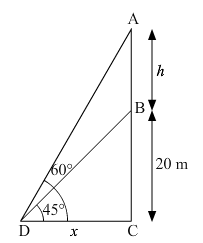# From a point on the ground the angles of elevation of the bottom and top of a communication tower fixed on the top of a 20-m-high building are 45°`
Question:

From a point on the ground the angles of elevation of the bottom and top of a communication tower fixed on the top of a 20-m-high building are 45° and 60° respectively. Find the height of the tower $[$ Take $\sqrt{3}=1.732]$

Solution:Let BC be the 20 m high building and AB be the communication tower of height h fixed on top of the building. Let D be a point on ground such that CD = x m and angles of

elevation made from this point to top and bottom of tower are $45^{\circ}$ and $60^{\circ}$

In $\triangle \mathrm{BCD}: \tan 45^{\circ}=\frac{\mathrm{BC}}{\mathrm{CD}}=\frac{20}{x}$

$\Rightarrow 1=\frac{20}{x} \Rightarrow x=20 \mathrm{~m}$

Also, in $\triangle \mathrm{ACD}: \tan 60^{\circ}=\frac{\mathrm{AC}}{\mathrm{CD}}=\frac{20+h}{x}$

$\Rightarrow \sqrt{3}=\frac{20+h}{x} \Rightarrow \sqrt{3}=\frac{20+h}{20}$

$\Rightarrow 20+h=20 \sqrt{3} \Rightarrow h=20 \sqrt{3}-20$

$\Rightarrow h=20(\sqrt{3}-1)=20(1.73-1)=20 \times 0.73$

$\Rightarrow h=14.64 \mathrm{~m} .$